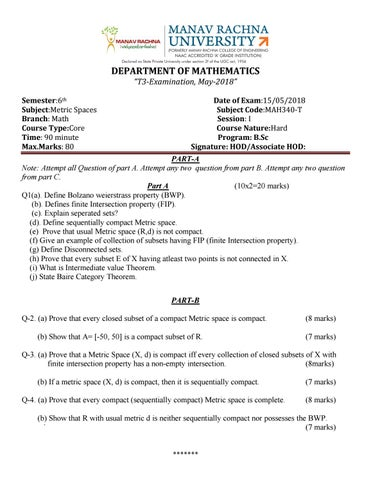DEPARTMENT OF MATHEMATICS â&#x20AC;&#x153;T3-Examination, May-2018â&#x20AC;? Semester:6th Subject:Metric Spaces Branch: Math Course Type:Core Time: 90 minute Max.Marks: 80

Date of Exam:15/05/2018 Subject Code:MAH340-T Session: I Course Nature:Hard Program: B.Sc Signature: HOD/Associate HOD:

PART-A Note: Attempt all Question of part A. Attempt any two question from part B. Attempt any two question from part C. Part A (10x2=20 marks) Q1(a). Define Bolzano weierstrass property (BWP). (b). Defines finite Intersection property (FIP). (c). Explain seperated sets? (d). Define sequentially compact Metric space. (e) Prove that usual Metric space (R,d) is not compact. (f) Give an example of collection of subsets having FIP (finite Intersection property). (g) Define Disconnected sets. (h) Prove that every subset E of X having atleast two points is not connected in X. (i) What is Intermediate value Theorem. (j) State Baire Category Theorem. PART-B Q-2. (a) Prove that every closed subset of a compact Metric space is compact. (b) Show that A= [-50, 50] is a compact subset of R.

(8 marks) (7 marks)

Q-3. (a) Prove that a Metric Space (X, d) is compact iff every collection of closed subsets of X with finite intersection property has a non-empty intersection. (8marks) (b) If a metric space (X, d) is compact, then it is sequentially compact. Q-4. (a) Prove that every compact (sequentially compact) Metric space is complete.

(7 marks) (8 marks)

(b) Show that R with usual metric d is neither sequentially compact nor possesses the BWP. ` (7 marks)

*******

PART-C Q-5. (a) If E is connected subset of a Metric space (X, d) such that đ??¸ â&#x160;&#x201A; đ??´ â&#x2C6;Ş đ??ľwhere A and B are Separated sets in X. Then prove that either Eâ&#x160;&#x201A;A or Eâ&#x160;&#x201A;B. (8 marks) (b) Let Eâ&#x160;&#x201A;(y, d*), a subspace of metric space (X, d). Then E is d*-connected iff E is d-connected. (8 marks) Q-6. (a) Prove that closure of a connected subset in (X, d) is also connected.

(8 marks)

(b) Let Eâ&#x160;&#x201A;(X, d). If any two points of E are contained in same connected subset of E Then E must be connected. (7marks) Q-7. (a) Prove that the set of real numbers with usual metric is a connected space. (8 marks) (b) Prove that every component of a metric space (X, d) is closed.

*******

(7 marks)